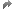Show TOC

# Expression BindingExpression binding is an enhancement of the SAPUI5 binding syntax, which allows for providing expressions instead of custom formatter functions.

This saves the overhead of defining a function and is recommended in cases where the formatter function has a trivial implementation like comparison of values. Expression binding is especially useful in the context of SAPUI5 XML templating where XML views with templating are preprocessed so that the SAPUI5 controller is a natural place to put custom formatter functions that are not available.

To use expression binding, you need to enable extended binding syntax via the configuration setting xx-bindingSyntax set to complex.

An expression binding is specified in an XML view by one of the following two options:

• {=expression}

This variant uses one-way binding. This allows the automatic recalculation if the model values change.

• {:=expression}

This variant uses one-time binding, meaning that the value is calculated only once. This variant needs less resources because no change listeners to the model have to be maintained.

expression complies to a subset of JavaScript expression syntax except for bindings embedded in the expression which are specified like this {=\${embedded}} To embed a path containing a closing curly brace into an expression binding, use a complex binding syntax: \${path:'...'}. Example: "{:= \${path:'target>extensions/[\${name} === \'semantics\']/value'} === 'email'}".

Besides embedded bindings, you can use the following:

Syntax Element

Symbol

Literal

number, for example 42, 6.022e+23 or -273.15

object, for example {foo: 'bar'}

string, for example 'foo'

null

true

false

Grouping

(...), for example 3 * (4 + 10)

Unary operator

!

+

-

typeof

Multiplicative operator

*

/

%

+

-

Relational operator

<

>

<=

>=

Strict equality operator

===

!==

Binary logical operator

&&

||

Conditional operator

?

Member access operator with the . operator

Note

With these, you can use members and member methods on standard types such as such as string, array, number, and so on.

Example: {\$message>/}.length}>0 or \${/firstName}.indexOf('S').

Function call

f(...)

Note

You can use functions that are available via global symbols, such as Math.max(...) or isNaN(...).

Array literals

[...], for example [2,3,5,7,11]

Property/array access

o[...], for example 'foo/bar'.split('/')

in operator

'PI' in Math (true) or 0 in [] (false)

Global symbol

Array, Boolean, Date, encodeURIComponent, Infinity, isFinite, isNaN, JSON, Math, NaN, Number, Object, parseFloat, parseInt, RegExp, String, undefined

Simple Example
Note

With expression binding you only need the XML view but no controller logic.

The following example shows how you use the custom formatter function to map an XML view to an expression binding in the XML view without controller logic.

The icon is only displayed if the status property in the view's default model has the value critical. You can use expression binding to replace the formatter function myFormatter in the controller with an expression binding in the XML view. You no longer need to implement any formatter function.

The application version without expression binding constists of the XML view (sample.App.view.xml) and the controller:

```<mvc:View controllerName="sample.App" xmlns="sap.ui.core" xmlns:mvc="sap.ui.core.mvc">
...
<Icon src="sap-icon://message-warning" visible="{path:'status', formatter:'.myFormatter'}">
...

</mvc:View>
```

Controller (sample.App.controller.js)

```...
myFormatter: function(sStatus) {
return sStatus === "critical";
}
...
```

When using expression binding, however, you only need the XML view without controller logic (sample.App.view.xml)

```<mvc:View controllerName="sample.app" xmlns="sap.ui.core" xmlns:mvc="sap.ui.core.mvc">
...
<Icon src="sap-icon://message-warning" visible="{= \${status} === 'critical' }">
...
</mvc:View>
```
More Complex Expressions

With the expression syntax sketched above it is possible to create more complex expressions as shown in the examples below.

Note

We recommend to use formatter functions instead of very complex and hard-to-read expressions. Some characters that are used by operators, however, need to be escaped in XML, for example the left angle bracket (<) and the ampersand (&). Escaping makes it more difficult to read the expression. To avoid escaping, use one of the following options:

• Rephrase the expression to make it more readable, for example, use a > b instead of b &lt; a.

• Use a custom formatter function.

For more information about escaping in XML, see the W3C XML specification at http://www.w3.org/TR/xml/#syntax.

Examples for more complex expressions:

```<!--Set to visible if the status is critical and the amount is above the threshold (note escaping of &&)-->
visible="{= \${status} === 'critical' &amp;&amp; \${amount} > 10000 }"```
```<!--Text for amount level using language-dependent texts from the resource model.-->
text="{= \${/amount} > 10000 ? \${i18n>/high} : \${i18n>/normal} }"```
```<!--Set to visible if the rating is VIP, ignoring case or if the order amount is greater than 10,000.-->
visible="{= \${/rating}.toUpperCase() === 'VIP' || \${/orderAmount} > 10000 }"```
```<!--Set to visible if the rating contains VIP, ignoring the case. -->
visible={= RegExp('vip', 'i').test(\${/rating}) }```
```<!--Text is maximum of three values.-->
text="{= Math.max(\${/value1}, \${/value2}, \${/value3}) }"```
```<!--Control is enabled only if the order status is set.-->
enabled="{= \${/orderStatus} !== null }"```
```<!--set text to the second string 'middle', access second element in the array generated via 'split'-->
text="{= 'small@middle@long'.split('@') }"```
```<!-- Concatenate literal strings and expression bindings or bindings -->
text="Hello {=\${gender}==='male' ? 'Mr.' : 'Mrs.'} {lastName}"```
```<!--Control such as a button in the toolbar of a table is enabled only if there are items in the table .-->
enabled="{= \${/items}.length>0 }"```